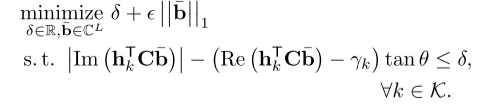# Unbounded problem for certan values of factor

#1

Hello everyone!

I want to implement the following minimization problem :Where

C is a NxL DFT Matrix.

Gamma and Theta are constants.

Epsilon is a positive weighting factor between 0…1 and should provide a certain level of sparsity of b.

I choosed to rewrite the problem in CVX using epsilon as weighting factor for both problems:

cvx_begin
variable b(4) complex;
variable delta;
minimize(delta*(1-epsilon) + epsilon*(norm(b,1)));
subject to
(abs(imag(H_sy.'*(C*b))) - (real(H_sy.'*(C*b))-y)*tano) - delta <=0;
cvx_end


Where H_sy is just a Matrix, containing the h_k vectors with length N. In my example, L is choosen to 4.

Now, for some values of epsilon (in my case 0…0.3 ) CVX does not find a solution and reports the following:

Calling SDPT3 4.0: 14 variables, 1 equality constraints
------------------------------------------------------------

num. of constraints =  1
dim. of socp   var  = 14,   num. of socp blk  =  5
*******************************************************************
SDPT3: Infeasible path-following algorithms
*******************************************************************
version  predcorr  gam  expon  scale_data
NT      1      0.000   1        0
it pstep dstep pinfeas dinfeas  gap      prim-obj      dual-obj    cputime
-------------------------------------------------------------------
0|0.000|0.000|0.0e+00|3.2e+00|2.8e+01| 2.792711e+00  0.000000e+00| 0:0:00| chol *   *
1|1.000|0.964|4.2e-17|2.1e-01|2.9e+00| 1.606495e+00  0.000000e+00| 0:0:00| chol *   *
2|1.000|0.192|1.0e-15|1.7e-01|9.0e-01|-3.724179e+00  0.000000e+00| 0:0:00| chol  1  1
3|1.000|0.018|1.2e-14|1.7e-01|2.0e+01|-9.298855e+03  0.000000e+00| 0:0:00| chol  1  1
4|1.000|0.002|2.2e-13|1.7e-01|4.6e+05|-2.331268e+09  0.000000e+00| 0:0:00| chol  1  1
stop: primal infeas has deteriorated too much, 4.0e+00
5|1.000|0.000|2.2e-13|1.7e-01|4.6e+05|-2.331268e+09  0.000000e+00| 0:0:00|
prim_inf,dual_inf,relgap = 2.17e-13, 1.67e-01, 1.99e-04
sqlp stop: dual problem is suspected of being infeasible
-------------------------------------------------------------------
number of iterations   =  5
residual of dual infeasibility
certificate X          = 9.32e-23
reldist to infeas.    <= 4.91e-24
Total CPU time (secs)  = 0.10
CPU time per iteration = 0.02
termination code       =  2
DIMACS: 2.2e-13  0.0e+00  2.0e-01  0.0e+00  -1.0e+00  2.0e-04
-------------------------------------------------------------------

------------------------------------------------------------
Status: Unbounded
Optimal value (cvx_optval): -Inf


So i have the following questions:

-I assume that my minimization problem is convex? … That holds true for every value of epsilon because epsilon is just a linear factor, right?
-Does the feasibility of the problem depend on the choice of epsilon?
-Or is there any other problem of my implementation?

Im a absolute beginner in CVX and convex problems - I apologize in case the answer is very obvious.

Best & thank you in advance!

Jul

(Mark L. Stone) #2

You haven’t provided the input data, so not a reproducible problem. For a given fixed (input) value of epilon, this is a convex optimization problem; although I defer judgment on whether it is a good formulation.

However my guess is that delta can go to -\infty along with
(real(H_sy.'*(C*b))-y)*tano) going to \infty, while satisfying the constraint, in such a way that delta*(1-epsilon) goes down faster than epsilon*(norm(b,1)) offsets it, hence making the problem (objective) unbounded. If that is the case, you need to rethink the model, the input data, or both.

#3

Thank you for your kind reply.

The following input data is used:

C = 1/sqrt(4)*exp(-1i*2*pi*(0:(4-1)).'*(0:(4-1))/4);

H = 1/sqrt(4)*(randn(4,2)+1i*randn(4,2));

s = [exp(j*(pi/4 + pi/4*2*randi([0 4-1], 1, 2)))];

H_sy = exp(1i*(angle(s(1,1))-angle(s(1,:)))).*H(:,:,1);

y = 0.7*ones(2,1)/sin(pi/4);

tano = tan(pi/4);

epsilon = 0.3;


Must i really review the model?

Maybe i have another problem in my implementation: not for every manipulating of epsilon a suitable value of norm(b,1) appears:

it should be as the following:

epsilon increasing -> norm(b,1) decreasing ; epsilon decreasing -> norm(b,1) increasing.

Or is this assumption wrong? (because maybe the delta plays in there?)

Thank you in advance, your answers would really help.

(Mark L. Stone) #4

You have not provided complete input data.

#5

Sorry,

the unbounded problem occurs for epsilons between 0…0.39 .
epsilon is a value between 0…1 .

(Mark L. Stone) #6

epsilon is not the only input data to your problem.

#7

ok, then i am a bit confusedi thought you meant variables… which kind of input data is missing?

thank you for your patience.

(Mark L. Stone) #9

Sorry, I misread a previous post. You did provide complete input data, other than that another user might not get the same random numbers as you, which cold have some effect. Indeed, the smallest value of epsilon for which a finite optimal solution exists does depend on the random numbers.

Nevertheless, my previous guess has been confirmed by actually running the problem.’
delta can go to −∞ along with
(real(H_sy.'*(C*b))-y)*tano) going to ∞, while satisfying the constraint, in such a way that delta*(1-epsilon) goes down faster than epsilon*(norm(b,1)) offsets it, hence making the problem (objective) unbounded.

Therefore, you need to rethink the model, the input data, or both. I have no idea what your problem is supposed to mean in “the real world”, nor is this a forum addressing the merits for various models, other than their implementation aspects.